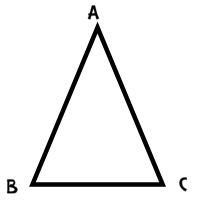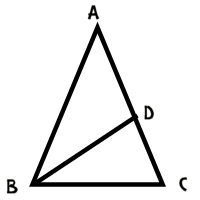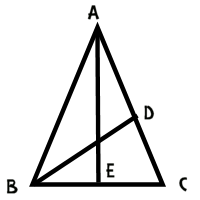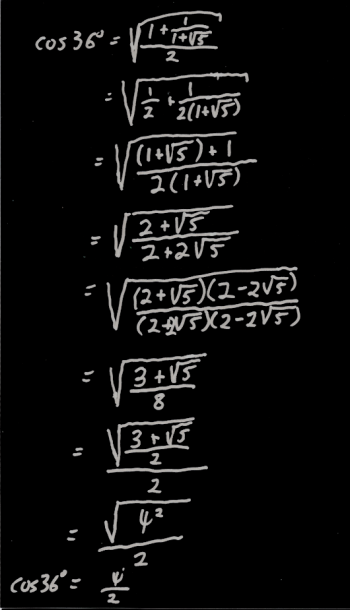Games
Problems
Go Pro!

Category results for 'proof'.

Bianca, an 11th grader from Canada asks, "Hello, I need help in math, how can I prove that the cosine of 36 is equal to the number of gold divided by 2."

That's a great question, Bianca. For anyone who doesn't know, the "number of gold" or "the golden ratio" is the number (1 + SQR(5))/2, which is approximately 1.6180339887. This number is interesting largely because the Greeks were fascinated by it, and because certain sequences which appear in nature sort of "zero in" on this number.

So how do we prove that the cosine of 36 degrees is this magical number? Well, we'll start by drawing a picture of an isosceles triangle which has angles 36-72-72.Let's add a line segment to this triangle; let's add an angle bisector from angle B to the opposite side.This produces some interesting similarities and congruences. For starters, measure of angle DBC is half of the measure of angle ABC, or 36º. Since the measure of angle DCB is 72º, we have triangle ABC similar to triangle BDC, and BC = BD (because the triangle is isosceles).

We can use some similar reasoning on triangle ABD to conclude that it is also an isosceles triangle, with BD = DA.

Putting all of this together leads to BC = BD = DA.

Now, since triangle ABD is similar to triangle BCD, we can conclude that

AC / BC = BC / CD

BC2 = (AC)(CD)

Now we can do a substitution, by noting that AC = AD + DC which equals BC + DC.

BC2 = (BC + DC)(DC)

BC2 = (BC)(DC) + DC2.

At this point, I'd like to do two things to this equation. First, I'd like to divide both sides by DC2, and then I'd like to do a substitution: x = BC/DC. This results in the following equation:

x2 - x - 1 = 0.

This can be solved using the quadratic formula: x = (1 + SQR(5))/2, or the golden ratio!

But how do we get here from to the trig function you want? Well, for starters, since the larger triangle is similar to the smaller one, we also have AB/BC = the golden ratio.Now if we draw a perpendicular down the middle of the diagram, we've got AB/BE = 1/2 of AB/BC, or half of the golden ratio. This means that BE/AB is 1/2 of the golden ratio. But BE/AB is the cosine of 72 degrees, since AEB is a right triangle.

Thus, cos(72º) = 2/x, where x is the golden ratio.

From here we can use a half angle identity:

cos(36º) = SQR((1 + cos(72º))/2)Now if you think that looks ugly, you're right. If you punch it into your calculator, you'll find that it evaluates to 0.809016994, which is half of the golden ratio. However, it would be nice to have an algebraic proof, right?

I worked through the algebra, but typing it all out on the blog would be UGLY, so I'm just going to post an image of the work I did on paper.

To view a larger version of this image, just click on it.

When I got to the end of the algebra, I had a radical within a radical, which is an ugly looking beast, but I happened to remember that (3 + SQR(5))/2 is the square of the golden ratio, which made the whole thing simplify quickly.

Is there an easier way to arrive at the cos(36º)? I'm sure there is. This was just the first one that came to mind. There might be other ways to do this using the law of cosines, or the law of sines, and I know you can do it with an entirely non-geometric proof (using various trig identities) but this one does the job. If you find a simpler proof, let me know!

"I noticed that all the perfect squares are either a multiple of 4, or they're one more than a multiple of 4. Is that a rule or something?" ~Wes in South Dakota

Wes, I don't know if it's a rule, exactly, but it's definitely a true statement. Every perfect square is either a multiple of four, or one more than a multiple of four. Would you like me to prove it?

Every integer is either even or odd. That means it's either a multiple of two, or it isn't. If it's a multiple of two, we can write it like this:

2n

If it's NOT a multiple of two, than it must be one more than a multiple of two. That means we can write it like this:

2n + 1

Every integer can be expressed either as 2n or as 2n + 1.

Therefore, every perfect square can be expressed as either (2n)2 or (2n + 1)2.

Now, if you've studied a bit of Algebra, you hopefully know how to simplify both of those expressions:

(2n)2 = 22n2 = 4n2

(2n + 1)2 = (2n + 1)(2n + 1) = 2n(2n + 1) + 1(2n + 1) = 4n2 + 2n + 2n + 1 = 4n2 + 4n + 1 = 4(n2 + n) + 1

Notice the result! The first possibility gives us a multiple of four, and the second one gives us one more than a multiple of four! And since that covers every perfect square, we can conclude that all perfect squares are either a multiple of four or one more than a multiple of four!

"Your blog post about palindromic squaring made me wonder - how many palindromic perfect squares are there?" ~D.

As a quick review for anyone who missed the post you referred to, a palindrome is a number (or word) that is the same forward and backward. For example 121 is a palindrome, because if you write it backwards, it's still 121.

So how many perfect squares are there that are palindromes? 121 is one example, but surely there must be others?

Yes. In fact, there are infinitely many perfect squares that are palindromes. Would you like a proof?

Let x = 10n + 1.

Then x2 = (10n + 1)(10n + 1)

x2 = 10n(10n + 1) + 1(10n + 1)

x2 = 102n + 10n + 10n + 1

x2 = 102n + 2·10n + 1

Written in base ten notation, this number would have 2n + 1 digits. The (n + 1)th digit would be 2, the first and last digits would be ones, and all the other digits would be zeroes. Since n+1 is halfway between 1 and 2n + 1 (note that n + 1 is the average of 1 and 2n + 1), the digits "mirror" each other, and the number is palindromic. This works for every positive integer n, which means  there are an infinite number of palindromic perfect squares. Just so you can see an example of this, let n = 4. Then we have:

x = 104 + 1 = 10001.

100012 = 100020001, which is a palindrome.

Of course, these all take the same form; a one at the beginning and end, a two in the middle, and everything else zero. You can find ones that take different forms:

2122 = 44944

1011012 = 10221412201

1111000011112 = 12343210246864201234321

And I submit (without proof) that for every form you find, you can create infinitely many palindromic perfect squares by inserting zeroes between the digits of the number you square.# Blogs on This SiteReviews and book lists - books we love!The site administrator fields questions from visitors.Like us on Facebook to get updates about new resources